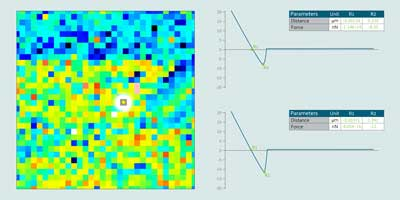Scroll Up

Stay up to-date with the latest imaging, analysis and metrology news from Digital Surf.

I agree to receive this newsletter and know that I can easily unsubscribe at any time.
Type the text you see :⇒ Automatic pre-processing of force curves – loaded force curves are automatically denoised (median or Gaussian filter), normalized (base-line correction) and calibrated (cantilever and spring constant settings).

⇒ Force curve visualization – display attract and/or retract curves – select axis units: X distance, separation or time; Y deflection (in V or nm) or force in nN.

⇒ Automatic event detection and parameter calculation – detect and display adhesion events automatically (with optional fine tuning of non-zero attraction point and retraction point cursors) – calculate event parameters: distance, force, slope, distance difference, force difference.

⇒ Wormlike chain (WLC) models – generate a wormlike chain (WLC) model for protein unfolding and calculate the persistence length, contour length and estimated spring constant at each event.

⇒ Analysis of series of force curves – grid view of all force curves in a series – scroll through a series of force curves and display parameters for each curve in the series – generate statistics (control charts, scatter plots, histograms) on any parameter.

Visualization of force volume data – display an interactive parameter map representing the value of a parameter calculated on each element of a force volume dataset.

⇒ Filter force volume and extract – smooth force volume data by removing noise and extract slices or individual force curves interactively.

Included in: